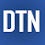## Pages

### Regression Example with AdaBoostRegressor in Python

Adaboost stands for Adaptive Boosting and it is widely used ensemble learning algorithm in machine learning. Weak learners are boosted by improving their weights and make them vote in creating a combined final model. In this post, we'll learn how to use AdaBoostRegressor class for the regression problem. AdaboostRegressor starts fitting the regressor with the dataset and adjusts the weights according to error rate. The tutorial covers:
1. Preparing data
2. Defining the model
3. Predicting and checking the accuracy

```from sklearn.ensemble import AdaBoostRegressor
from sklearn.model_selection import train_test_split
from sklearn.model_selection import cross_val_score, KFold
from sklearn.metrics import mean_squared_error
import matplotlib.pyplot as plt```

Preparing data

We use Boston house-price dataset as regression dataset in this tutorial. After loading the dataset, first, we'll separate data into x - feature and y - label. Then we'll split them into the train and test parts. Here, I'll extract 15 percent of the dataset as test data.

```boston = load_boston()
x, y = boston.data, boston.target
xtrain, xtest, ytrain, ytest=train_test_split(x, y, test_size=0.15)```

Defining the model

We'll define the model with AdaBoostRegressor class. Here, we'll set 100 estimators and keep the other parameters as they are.

```ada_reg = AdaBoostRegressor(n_estimators=100)
```AdaBoostRegressor(base_estimator=None, learning_rate=1.0, loss='linear',
n_estimators=100, random_state=None) ```

Then, we'll fit the model with a train and test data

`ada_reg.fit(xtrain, ytrain)`

Predicting and checking the accuracy

After training the model, we can check the accuracy with the cross-validation method.

```scores = cross_val_score(ada_reg, xtrain,ytrain,cv=5)
print("Mean cross-validataion score: %.2f" % scores.mean())```
`Mean cross-validataion score: 0.77 `

We can also apply cross-validation with a k-fold method.

```kfold = KFold(n_splits=10, shuffle=True)
kf_cv_scores = cross_val_score(ada_reg, xtrain, ytrain, cv=kfold )
print("K-fold CV average score: %.2f" % kf_cv_scores.mean())```
`K-fold CV average score: 0.82 `

Next, we'll predict test data and check its accuracy. Here, we'll use MSE and RMSE accuracy metrics.

```ypred = ada_reg.predict(xtest)
mse = mean_squared_error(ytest,ypred)
print("MSE: %.2f" % mse)```
`MSE: 15.82 `
`print("RMSE: %.2f" % np.sqrt(mse))`
`RMSE: 3.98  `

Finally, we'll visualize the original and predicted test data in a plot.

```x_ax = range(len(ytest))
plt.scatter(x_ax, ytest, s=5, color="blue", label="original")
plt.plot(x_ax, ypred, lw=0.8, color="red", label="predicted")
plt.legend()
plt.show()
```

In this post, we've briefly learned how to use AdaBoostRegressor to predict regression data in Python. Thank you for reading.
The full source code is listed below.

```from sklearn.ensemble import AdaBoostRegressor
from sklearn.model_selection import train_test_split
from sklearn.model_selection import cross_val_score, KFold
from sklearn.metrics import mean_squared_error
import matplotlib.pyplot as plt

x, y = boston.data, boston.target
xtrain, xtest, ytrain, ytest=train_test_split(x, y, test_size=0.15)

### - cross validataion
print("Mean cross-validataion score: %.2f" % scores.mean())

# k-fold cross validataion
kfold = KFold(n_splits=10, shuffle=True)
kf_cv_scores = cross_val_score(ada_reg, xtrain, ytrain, cv=kfold )
print("K-fold CV average score: %.2f" % kf_cv_scores.mean())

# prediction
mse = mean_squared_error(ytest,ypred)
print("MSE: %.2f" % mse)
print("RMSE: %.2f" % np.sqrt(mse))

# plotting the result
x_ax = range(len(ytest))
plt.scatter(x_ax, ytest, s=5, color="blue", label="original")
plt.plot(x_ax, ypred, lw=0.8, color="red", label="predicted")
plt.legend()
plt.show()```

1.1.